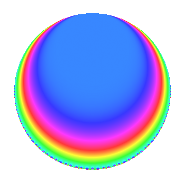# Properties

 Label 3600.1.e.aLevel 3600 Weight 1 Character orbit 3600.e Self dual Yes Analytic conductor 1.797 Analytic rank 0 Dimension 1 Projective image $$D_{2}$$ CM/RM disc. -4, -20, 5 Inner twists 4

# Related objects

## Newspace parameters

 Level: $$N$$ = $$3600 = 2^{4} \cdot 3^{2} \cdot 5^{2}$$ Weight: $$k$$ = $$1$$ Character orbit: $$[\chi]$$ = 3600.e (of order $$2$$ and degree $$1$$)

## Newform invariants

 Self dual: Yes Analytic conductor: $$1.79663404548$$ Analytic rank: $$0$$ Dimension: $$1$$ Coefficient field: $$\mathbb{Q}$$ Coefficient ring: $$\mathbb{Z}$$ Coefficient ring index: $$1$$ Projective image $$D_{2}$$ Projective field Galois closure of $$\Q(i, \sqrt{5})$$ Artin image size $$8$$ Artin image $D_4$ Artin field Galois closure of 4.2.18000.1

## $q$-expansion

 $$f(q)$$ $$=$$ $$q + O(q^{10})$$ $$q + 2q^{29} + 2q^{41} + q^{49} - 2q^{61} + 2q^{89} + O(q^{100})$$

## Character Values

We give the values of $$\chi$$ on generators for $$\left(\mathbb{Z}/3600\mathbb{Z}\right)^\times$$.

 $$n$$ $$577$$ $$901$$ $$2801$$ $$3151$$ $$\chi(n)$$ $$1$$ $$1$$ $$1$$ $$-1$$

## Embeddings

For each embedding $$\iota_m$$ of the coefficient field, the values $$\iota_m(a_n)$$ are shown below.

For more information on an embedded modular form you can click on its label.

Label $$\iota_m(\nu)$$ $$a_{2}$$ $$a_{3}$$ $$a_{4}$$ $$a_{5}$$ $$a_{6}$$ $$a_{7}$$ $$a_{8}$$ $$a_{9}$$ $$a_{10}$$
3151.1
 0
0 0 0 0 0 0 0 0 0
 $$n$$: e.g. 2-40 or 990-1000 Significant digits: Format: Complex embeddings Normalized embeddings Satake parameters Satake angles

## Inner twists

Char. orbit Parity Mult. Self Twist Proved
1.a Even 1 trivial yes
4.b Odd 1 CM by $$\Q(\sqrt{-1})$$ yes
5.b Even 1 RM by $$\Q(\sqrt{5})$$ yes
20.d Odd 1 CM by $$\Q(\sqrt{-5})$$ yes

## Hecke kernels

This newform can be constructed as the intersection of the kernels of the following linear operators acting on $$S_{1}^{\mathrm{new}}(3600, [\chi])$$:

 $$T_{7}$$ $$T_{13}$$